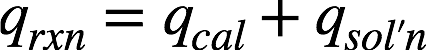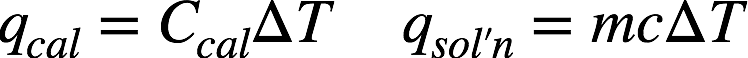# Problem: When a 0.740 g sample of trinitrotoluene (TNT), C 7H5N2O6, is burned in a bomb calorimeter, the temperature increases from 23.4°C to 26.9°C. The heat capacity of the calorimeter is 534 J/°C, and it contains 675 mL of water. How much heat was produced by the combustion of the TNT sample?

###### FREE Expert Solution

Recall: In a bomb calorimeter, the heat of a reaction is given by:where qcal = heat absorbed by the calorimeter and qsol'n = heat absorbed by water in the calorimeter. The respective equations for the two are as follows:where Ccal = heat capacity of the calorimeter (in J/˚C), m = mass of solution (in g), c = specific heat of solution (in J/g•˚C), and ∆T = change in temperature (final T – initial T, in ˚C).

90% (309 ratings)###### Problem Details

When a 0.740 g sample of trinitrotoluene (TNT), C 7H5N2O6, is burned in a bomb calorimeter, the temperature increases from 23.4°C to 26.9°C. The heat capacity of the calorimeter is 534 J/°C, and it contains 675 mL of water. How much heat was produced by the combustion of the TNT sample?